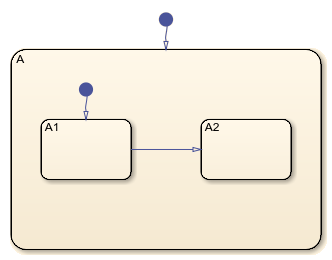# getParent

Identify parent of object

## Syntax

``parent = getParent(object)``

## Description

example

````parent = getParent(object)` returns the parent of an object in a Stateflow® chart, State Transition Table, Truth Table, or MATLAB® Function block.```

## Examples

collapse all

Suppose that `ch` is the `Stateflow.Chart` object that corresponds to this chart. In this chart, the parent of state `A1` is state `A`. The parent of state `A` is the chart.Find the `Stateflow.State` object named `A1`.

`sA1 = find(ch,"-isa","Stateflow.State",Name="A1");`

Identify the parent of state `A1`. Display the name of the parent.

```parent = getParent(sA1); parent.Name```
```ans = 'A'```

Identify the parent of state `A`. Display the name of the parent.

```grandparent = getParent(parent); grandparent.Name```
```ans = 'Chart' ```

## Input Arguments

collapse all

Object in a Stateflow chart, State Transition Table, Truth Table, or MATLAB Function block, specified as a Stateflow API object of one of these types:

## Version History

Introduced before R2006a